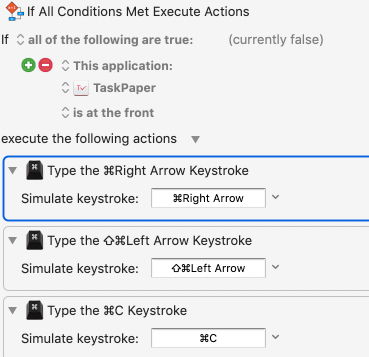# Add a hotkey to copy current line

I would love if there was a hotkey added to copy to clipboard the current active line in TaskPaper.

Right now I am forced to emulate three keystrokes to do that:And I really dislike that.

Thank you.

For something like an Execute JavaScript action in Keyboard Maestro, you could use a script along these lines:

( In the withOptions object, change the textProperty value from `bodyString` to `bodyContentString` if you don’t want to copy the Taskpaper bullet-hyphens, colons and tags )

``````(() => {
'use strict';

const main = () => {

const tp3Context = (editor, options) => {

const main = () =>
unlines(
concatMap(
x => {
const txt = x[options.textProperty];
return options.skipBlankLines && 0 ===
txt.length ? (
[]
) : [txt];
},
editor.selection.selectedItems
)
);

// GENERIC FUNCTIONS FOR TP3 CONTEXT ----------
// https://github.com/RobTrew/prelude-jxa

// concatMap :: (a -> [b]) -> [a] -> [b]
const concatMap = (f, xs) =>
xs.reduce((a, x) => a.concat(f(x)), []);

// unlines :: [String] -> String
const unlines = xs => xs.join('\n');

// TP3 MAIN ---
return main();
};

const
.documents,
lrResult = bindLR(
ds.length > 0 ? (
Right(ds.at(0))
) : Left('No TaskPaper documents open'),
d => Right(d.evaluate({
script: tp3Context.toString(),
withOptions: {
skipBlankLines: true,
textProperty: 'bodyString' // or 'bodyContentString'
}
}))
);
return lrResult.Left || (
lrResult.Right
);
};

// GENERIC FUNCTIONS FOR JXA CONTEXT ------------------
// https://github.com/RobTrew/prelude-jxa

// Left :: a -> Either a b
const Left = x => ({
type: 'Either',
Left: x
});

// Right :: b -> Either a b
const Right = x => ({
type: 'Either',
Right: x
});

// bindLR (>>=) :: Either a -> (a -> Either b) -> Either b
const bindLR = (m, mf) =>
undefined !== m.Left ? (
m
) : mf(m.Right);

// MAIN ----
return main();
})();

``````
1 Like

See:

⌃S (Control-S) with ⌘C should do what you want in two keystrokes.

Of course, Keyboard Maestro can make this into one keystroke.

Works perfectly. Thank you.

1 Like